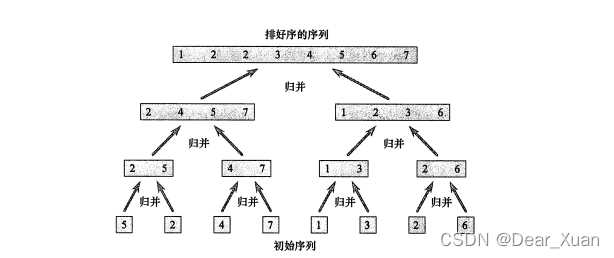## 算法

### 为什么要用算法

• 为了走出迷宫,你可能需要DFS,即深度优先搜索算法来寻找出路.
• 为了找到最短路径,你可能要用到A*算法来高效查找.
• 为了将一串数字排序,你需要用更快的快速排序算法,而不是一个一个比较.

## 分治法

### 归并排序### 时间复杂度

$T(n)= \left \{ \begin{array}{ll} O(1) &,n=1 \\\\ 2T\left(\frac n2\right) &,n > 1 \end{array} \right.$

$\begin{array}{ll} T(n) &=2T(\frac n2)+O(n) \\\\ &=2^2T(\frac n4)+2O(n) \\\\ &=... \\\\ &=2^kT(\frac n{2^k})+kO(n) \end{array}$

$\begin{array}{ll} T(n) &=2T(\frac n2)+O(n) \\\\ &=2^2T(\frac n4)+2O(n) \\\\ &=... \\\\ &=2^kT(\frac n{2^k})+kO(n) \\\\ &=nO(1)+(\log n)O(n) \\\\ &=O(n\log n) \end{array}$